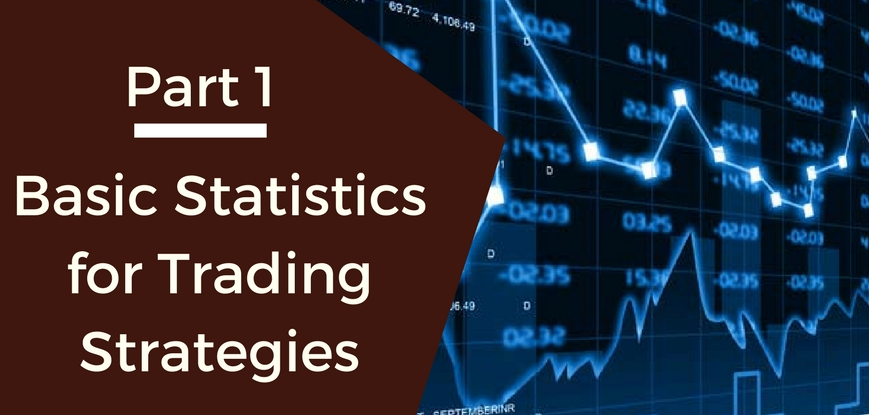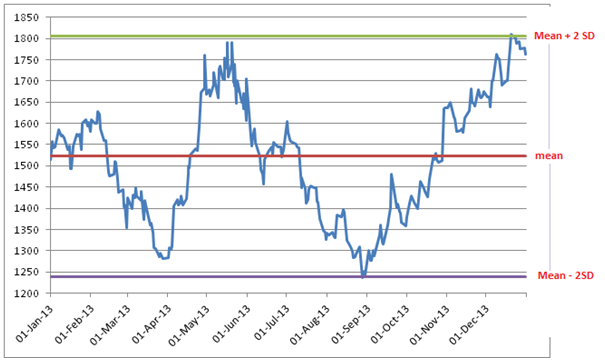# Basic Statistics for Trading Strategies (Part 1) - Historical Data AnalysisThis series of posts is to get our readers to start using statistics and data analysis while trading. This post tries to answer the basic question: “how do you analyze stock’s historical data and use it for strategy building?” In this post, we will focus on using Excel to do the analysis. In the subsequent post(s), We will go through the basic codes in R which you can use to try out the similar analysis in R.

### Dataset

MARUTI SUZUKI India Limited- Daily data from Jan 01, 2013 to Dec 31, 2013. Public link to download the corresponding dataset: http://nseindia.com/products/content/equities/equities/eq_security.htm

This is a time series data set with daily closing prices and volumes for Maruti. We’ll base our analysis on the closing prices for this stock. We’ll just touch upon the very basic statistical properties for the daily stock prices in this post, which would be followed by calculating beta and a simple moving average for this stock later.

### Mean

Mean is the average of a data set. The average of the daily closing price for Maruti in 2013 was 1522.47. However, for the last day of the year, 31-Dec-2013, the closing price was 1763.9. In Excel, you can use the function to calculate the mean.

### Mode

Mode gives us the most frequently occurring value in the dataset. This measure doesn’t really make much sense for closing prices or other continuous data. A mode is especially useful when you want to plot histograms and visualize the frequency distribution. In Excel, you can calculate the mode for a given dataset using a function.

### Median

Median gives the middle value of the increasingly sorted data set. It is especially useful in circumstances when the dataset has a few values at the extreme ends. For example, the salary in a company might vary between Rs. 2000 to Rs. 1,00,00,000. The mean would be influenced by a few extreme situations and might get skewed negatively or positively. To avoid this, a median is used which gives the middle point of the distribution. In Excel, you can calculate median for a given dataset using a function.

### Standard Deviation

Volatility of the stock can be calculated using standard deviation. The volatility of Maruti stock in given period is 141.26. The standard deviation can be for the population as well as for the sample. In quantitative trading strategy modeling, you’ll be using sample standard deviation most of the times. Excel formula for calculating the sample standard deviation is.

### Range

Range simply gives the difference between the min and max values of the data set. Minimum and maximum values for Maruti closing prices in 2013 are 1236.35 and 1809.65. However, it makes more sense to consider the intraday high and low as well to get the high and low for the stock in a year which gives us the range as 1126 to 1830.

The graph below shows all the measures in a graph so that you get a sense of all the values.You can notice how the interval (mean -2SD, mean + 2SD) captures most of the data points. Statistically speaking 95.46% data is within that interval assuming a normal distribution.

Moving average and standard deviations are used to create Bollinger bands too, which is one of the many ways of predicting the range for the stock price technical analysis and you can also form various trading strategies based on Bollinger bands.

### Next Step

In part 2 of this series, we will try to understand distributions and will also try to answer the basic question: “Why is statistics necessary for strategy building?”

In part 3 of this series, we will try to understand the relationship between a stock and a market index. The terms we will understand are regression, correlation and cointegration.

You can also refer our post on 'Build Technical Indicators In Python'  that explains how to create python technical indicators which are popularly used by technical analysts in the markets to study the price movement.

Disclaimer: All data and information provided in this article are for informational purposes only. QuantInsti® makes no representations as to accuracy, completeness, currentness, suitability, or validity of any information in this article and will not be liable for any errors, omissions, or delays in this information or any losses, injuries, or damages arising from its display or use. All information is provided on an as-is basis.